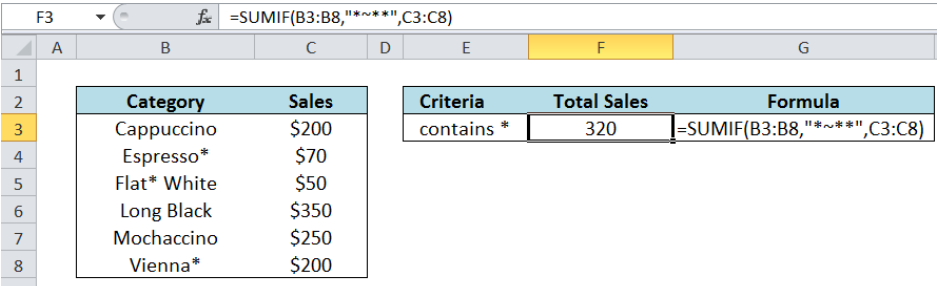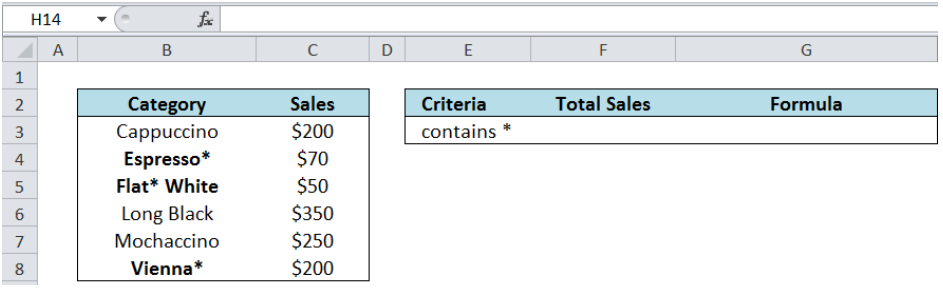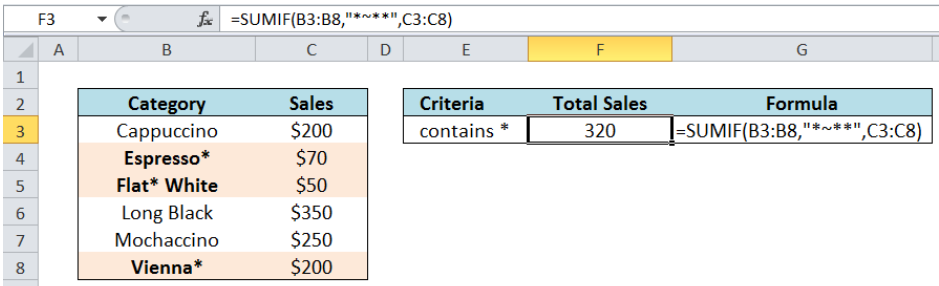Get instant live expert help with Excel or Google Sheets“My Excelchat expert helped me in less than 20 minutes, saving me what would have been 5 hours of work!”

#### Post your problem and you’ll get Expert help in seconds.

Your message must be at least 40 characters
Our professional Expert are available now. Your privacy is guaranteed.

# Sum if cells contain an asterisk

Summing cells that contain an asterisk is tricky in Excel because the asterisk *” is a wildcard.  In Excel, it means any string of characters. This article will teach us how to sum cells containing an asterisk using SUMIF and the tilde (~).Figure 1.  Final result: Sum if cells contain an asterisk

The tilde ~ is also a wildcard in Excel.  It is used to mark the character next to it as a literal character.

## SUMIF function in Excel

SUMIF sums the values in a specified range, based on one given criteria

### Syntax

`=SUMIF(range,criteria, [sum_range])`

Where

• Range: the data range that will be evaluated using the criteria
• Criteria: the criteria or condition that determines which cells will be added
• Sum_range: the cells that will be added; if left blank, “sum_range” = “range” which means that the range of data that will be added is the same range of data evaluated

## Setting up the Data

Let’s create a list of categories and sales for coffee.  We want to sum the sales for categories containing an asterisk *”.Figure 2.  Sample data to sum cells containing an asterisk

## Sum Cells Containing an Asterisk

In cell F3, enter the formula:

`=SUMIF(B3:B8,"*~**",C3:C8)`

Where

• B3:B8 is the range of data we want to evaluate, which is under column B “Category”
• C3:C8 is the range whose values we want to add, once the criteria is satisfied in column B
• The formula returns the value 320, which is the sum of sales for Espresso*, Flat* White and Vienna*Figure 3.  Entering the formula using SUMIF, tilde ~ and asterisk *

## Important Notes

• *~**” is the criteria; the asterisks in both sides ensure to include all cells containing an asterisk, no matter where the asterisk is in a cell.
• Example: In “ Espresso* ”, the asterisk is the last character but in “ Flat* White ”, the asterisk is in the middle.
• Note the characters inside the two asterisks, ~* : it is necessary to put the ~ before the asterisk “*” in order for Excel to search for the asterisk “*” as a literal character, and not as a wildcard

Most of the time, the problem you will need to solve will be more complex than a simple application of a formula or function. If you want to save hours of research and frustration, try our live Excelchat service! Our Excel Experts are available 24/7 to answer any Excel question you may have. We guarantee a connection within 30 seconds and a customized solution within 20 minutes.

### Did this post not answer your question? Get a solution from connecting with the expert.Another blog reader asked this question today on Excelchat:## Subscribe to Excelchat.coAnother blog reader asked this question today on Excelchat: